Next: Dirac Particle at Rest Up: Dirac Equation Previous: Wave Equation for a

# Current Conservation

The hermitian conjugate wave function is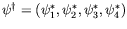. Multiplying the Dirac equation by the conjugate wave function from the left gives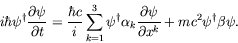(5.11)

Forming the Dirac equation for the conjugate wave function and multiplying by the wave function from the right gives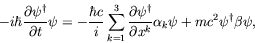(5.12)

where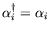and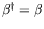. Subtracting (5.11) from (5.12) gives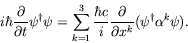(5.13)

Writing the result as a continuity equation,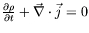, gives the probability density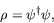(5.14)

and the probability current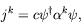(5.15)

where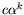is like a velocity operator.

Integrating over all space and using the divergence theorem gives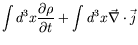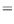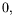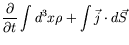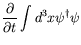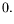(5.16)

We have now proven the first two conditions required of the Dirac equation but we still have to show (c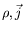) forms a 4-vector under a Lorentz transformation and that the Dirac equation is Lorentz covariant.Next: Dirac Particle at Rest Up: Dirac Equation Previous: Wave Equation for a
Douglas M. Gingrich (gingrich@ ualberta.ca)
2004-03-18